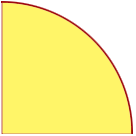SEARCH HOMEMath Central Quandaries & QueriesQuestion from Bethany, a student: The perimeter of a quarter circle is 3.57. What is the quarter circle's radius?Hi Bethany,

The perimeter of a quarter circle is composed of three pieces, two are straight and are radii of the circle and the third piece is curved and is one-quarter of the circumference (perimeter) of the circle.Suppose the radius of the circle is $r$ units. The perimeter of the quarter circle is then $r + r + \mbox{ one-quarter the circumference of the circle.}$ What is the expression for the circumference of a circle of radius $r$ units. Solve for $r.$

PennyMath Central is supported by the University of Regina and the Imperial Oil Foundation.